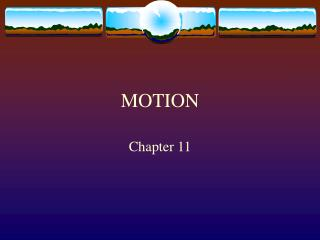# MOTION - PowerPoint PPT PresentationDownload PresentationMOTION

MOTION
Download Presentation## MOTION

- - - - - - - - - - - - - - - - - - - - - - - - - - - E N D - - - - - - - - - - - - - - - - - - - - - - - - - - -
##### Presentation Transcript

1. MOTION Chapter 11

2. How do you know something is moving? • Depends on your frame of reference. • A frame of reference is the location from which motion is observed. • Most common: the earth.

3. Measuring distance • Distance: the length of a path between two points. • Considered a scalar quantity. (only a magnitude) • Can be expressed in any number of units. • Choose a unit that is best suited for the motion being described. • Examples: meters, kilometers, centimeters

4. Measuring displacement • Displacement is independent of the path taken by the object. • Involves the starting point, ending point, anddirection.Vector quantity • Example:

5. Adding vectors • When the vectors are along the same plane they are added arithmetically. • Many different methods for adding vectors that do not lie in the same plane. • Examples on board

6. What is SPEED ? • The ratio of distance an object moves to the amount of time it takes to move that distance. • How fast or slow an object is traveling. • Is speed a vector or a scalar quantity scalar

7. Average Speed • Defined as the total distance / total time. Or •  = d/t • Example problems on page 333

8. Instantaneous speed • Not as useful in physical science. Much more difficult to calculate. • Defined as the speed at a given moment in time.

9. Graphing motion • Distance-time graphs. • The slope of a line on a distance-time graph is the speed. • At what speed is the student walking down the hallway?

10. Speed vs velocity • Velocity is speed in a given direction. • MUST have a direction. • Considered a vector quantity

11. What is acceleration? • A situation in which the velocity is changing. • “Speeding up or slowing down” • “changing direction” • Science definition: the rate of change of velocity • Can be both negative and positive • Acceleration is a VECTOR quantity.

12. Acceleration examples: • Which car is experiencing acceleration?

13. If you said the red and blue car you were right! • A distance-time graph of all three cars: • The distance-time graph for accel. Is always a curve.

14. What is the formula for acceleration? • Acceleration = final velocity – original velocity time Or the change in velocity ( v) / change in time (t) • Label : m/sec/sec or m/sec2

15. Example problems: • A roller coaster’s velocity at the top of a hill is 10 m/sec. Two seconds later it reaches the bottom of the hill with a velocity of 26 m/sec. What is the acceleration of the roller coaster?

16. More examples: • A roller coaster is moving 25 m/sec at the bottom of a hill. Three seconds later it reaches the top of the hill and is traveling at a speed of of 10 m/sec. What was the roller coaster’s acceleration? • Can you have negative acceleration? • YES. The negative sign tells us the direction of the acceleration, usually means deceleration.

17. Free Fall • Acceleration due solely to the force of gravity. • All objects fall at the same rate. • 9.8 m/sec2

18. Graphing acceleration • Distance-time graph for constant acceleration always a CURVE. • Velocity-time graph for constant acceleration always a STRAIGHT LINE.

19. Example graphs:

20. Assignment: • Pages 351-353 • 1-10, 17,18,19,20,21,24,29,30 • 1-5 page 353